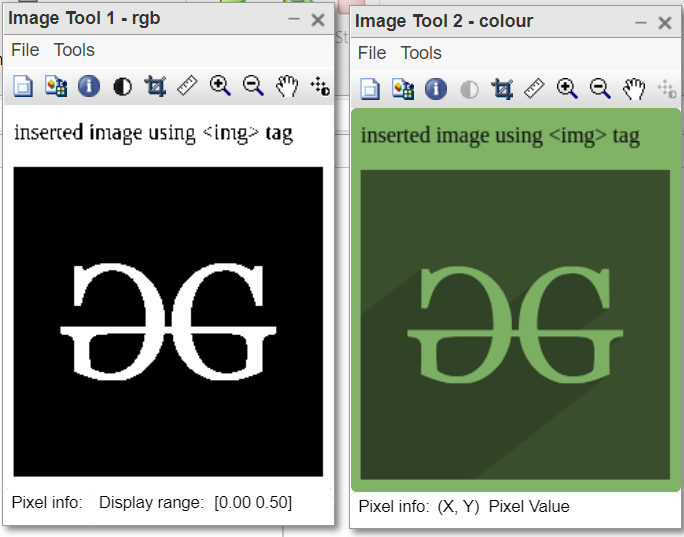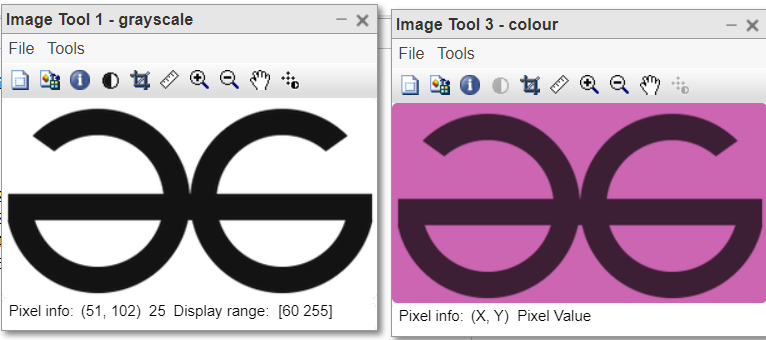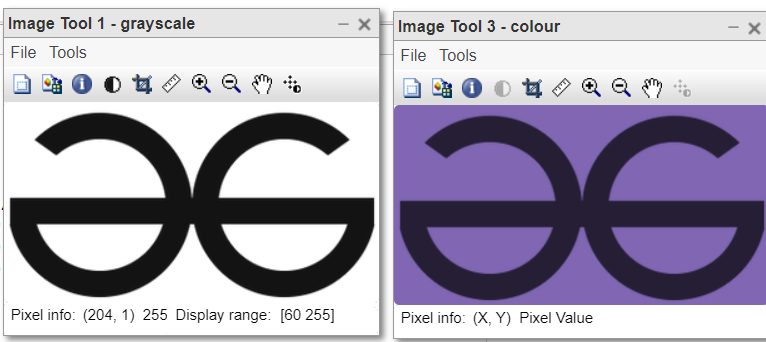Open In App

# How to Perform Random Pseudo Coloring in Grayscale Image Using MATLAB?

Pseudo Coloring is one of the attractive categories in image processing. It is used to make old black and white images or videos colorful. Pseudo Coloring techniques are used for analysis identifying color surfaces of the sample image and adaptive modeling of histogram black and white image. Selecting different values in the layers R, G, B is the most important achievement of this technique such that this method is based on the analysis of histogram characteristics in the sample image, to assign different values in different layers of a color image, we take action. Pseudo-coloring is also known as a coloring topic in processing digital images.

In this technique, a gray level of type integer unsigned 8-bit (a number between zero and 255) should be considered as input and three outputs must be achieved for three layers of the color digital image graph and each of these three levels should be of type integer unsigned 8-bit (a number between zero and 255). There are many techniques for this conversion that have some differences based on our needs.

####• Grayscale image: It is a black and white image. The pixels values are shades of gray colour which is the combination of white and black shades. The image is represented in form of one 2-Dimensional matrix. Each value represents the intensity or brightness of the corresponding pixel at that coordinate in the image. Total 256 shades are possible for the grayscale images. 0 means black and 255 means white. As we increase the value from 0 to 255, the white component gets increases and brightness increases.
• RGB color image: It is a colored image. It consists of three 2-Dimensional matrices, which are called channels. Red, Green and Blue channels contain the corresponding colour values for each pixel in the image. In integer format, the range of pixel intensity goes from 0 to 255. 0 means black and 255 represents the highest intensity of the primary colour. There exist 256 shades of each colour.

#### Steps:

• If its bit-depth is 24, then make it 8.
• Create an empty image of the same size.
• Assign some random weight to RGB channels.
• Copy weighted product of grayscale image to each channel of Red, Green, and Blue.
• Display the images after creation.

Functions Used:

• imtool( ) inbuilt function is used to display the image.
• rgb2gray( ) inbuilt function is used to convert RGB to gray image.
• uint8( ) inbuilt function is used to convert double into integer format.
• pause( ) inbuilt function is used to stop execution for specified seconds.

Example:

## Matlab

 `% MATLAB code for pseudo colouring``% of grayscale images.``% UTILITY CODE``k=imread(``"gfglogo.png"``);``gray2rgb(k);``imtool(grayscale,[]);``function` `gray2rgb(img)`` ` `% Convert into grayscale if not.``[x,y,z]=size(img);``if``(z==3)``    ``grayscale=rgb2gray(img);``end``gray=double(grayscale./255);``rgb(:,:,1)=gray(:,:)*0.5;``rgb(:,:,2)=gray(:,:)*0.6;``rgb(:,:,3)=gray(:,:)*0.4;``imtool(rgb,[]);`` ` `c(x,y,z)=0;``colour=uint8(c);``colour(:,:,1)=grayscale(:,:)*0.5;``colour(:,:,2)=grayscale(:,:)*0.7;``colour(:,:,3)=grayscale(:,:)*0.4;``imtool(colour,[]);`` ` `pause(10);``imtool close all;``end`

Output:Code Explanation:

• [x,y,z]=size(img); This line gets the size of input image.
• gray=double(grayscale./255); This line converts input image into double format.
• rgb(:,:,1)=gray(:,:)*0.5; This line builds red channel.
• rgb(:,:,2)=gray(:,:)*0.6; This line builds green channel.
• rgb(:,:,3)=gray(:,:)*0.4; This line builds blue channel.
• imtool(rgb,[]); This line displays the build Plain – RGB image.
• c(x,y,z)=0; This line creates empty image with black pixels.
• colour=uint8(c); This line converts image into integer format.
• colour(:,:,1)=grayscale(:,:)*0.5; This line populates the red channel.
• colour(:,:,2)=grayscale(:,:)*0.7; This line populates the green channel.
• colour(:,:,3)=grayscale(:,:)*0.4; This line populates the blue channel.
• imtool(colour,[]); This line displays the coloured image formed.
• pause(10); This line halts the execution for 10 seconds.